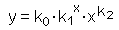The SDL Component Suite is an industry leading collection of components supporting scientific and engineering computing. Please visit the SDL Web site for more information....## CalcHoerlFitUnit: SDL_math2 Class: Declaration: procedure CalcHoerlFit (var k0, k1, k2, FitQual: double);

The procedure CalcHoerlFit calculates the best fitting generalized Hoerl equation for a given set of data. The curve is determined by the equationThe values of x and y are given by the data samples, the parameters k0 and k1 are estimated by CalcHoerlFit using a least squares approximation. An exception is raised if the fit cannot be calculated (i.e. if the x-values are too large).

The data points [x,y] have to be entered using the routine EnterStatValue. A minimum number of 3 values is required in order to apply CalcHoerlFit. Do not forget to reset the statistics calculation before entering any new data sets (use the method Init)

In addition to the parameters k0 and k1 CalcHoerlFit the quality of fit FitQual. This parameter may vary between 0.0 and 1.0, indicating the best possible fit if FitQual equals 1.0.

 Hint 1: The quality of fit calculated by CalcHoerlFit is not adjusted for the degree of freedoms in the regression parameters.

 Hint 2: Both the x- and the y-values have to be greater than zero. The curve fitting algorithm raises an exception in the case of negative or zero values.

 Example: This method is used in the following example program (see http://www.lohninger.com/examples.html for downloading the code): curvefit

Last Update: 2012-Okt-20